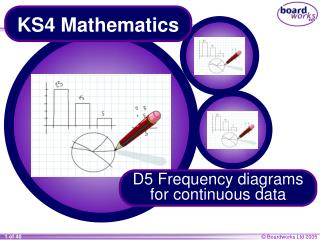DownloadDownload PresentationKS4 Mathematics

# KS4 Mathematics

Télécharger la présentation## KS4 Mathematics

- - - - - - - - - - - - - - - - - - - - - - - - - - - E N D - - - - - - - - - - - - - - - - - - - - - - - - - - -
##### Presentation Transcript

1. KS4 Mathematics D5 Frequency diagrams for continuous data

2. D5 Frequency diagrams for continuous data Contents D5.1 Grouping continuous data • A D5.2 Frequency diagrams • A D5.4 Histograms D5.3 Frequency polygons • A • A D5.5 Frequency density • A

3. 12 10 8 Frequency 6 4 2 0 95 100 105 110 115 120 125 130 135 Time in seconds Histograms This frequency diagram represents the race times for the Youth category, which is 14 to 16 year olds. There are __ times as many people in the 105 ≤t < 110 interval than there are in the 95 ≤ t < 100 interval. 3 Is the bar three times as big? How many people are represented by each square on the grid?

4. 12 10 8 Frequency 6 4 2 0 95 100 105 110 115 120 125 130 135 Time in seconds Histograms Discuss this statement. Do you agree or disagree? “If a bar is twice as high as another, the area will be twice as big and so the frequency will be twice the size.”

5. 12 10 8 Frequency 6 4 2 0 95 100 105 110 115 120 125 130 135 Time in seconds Combining intervals Some of the intervals are very small, which makes any conclusions about them unreliable. It is sometimes sensible to combine intervals together. Which intervals would you combine?

6. 12 10 8 Frequency 6 4 2 0 95 100 105 110 115 120 125 130 135 Time in seconds Histograms with bars of unequal width This graph represents the same data as the previous one. What has changed? The first two intervals both had a frequency of 2. The first bar now represents an interval twice as big. How many people are in this interval? How many people does one square represent? Do the numbers along the vertical axis still represent frequency?

7. Frequency 0 95 100 105 110 115 120 125 130 135 Time in seconds Histograms with bars of unequal width In the original histogram, the frequency was proportional to the area. Is this still true in the new histogram? The frequency for 105 ≤t < 110 is the same as the frequency for ___________. 120 ≤t < 135 Are the areas of the bars the same? Each square stills represents two people. In a histogram, the frequency is equal to the area of the bar.